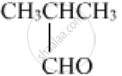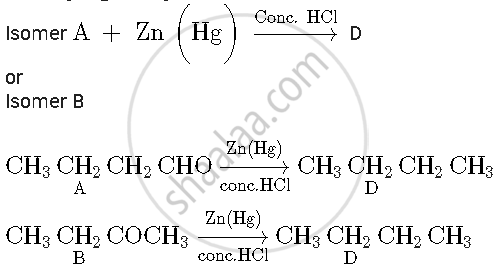Share

# (A), (B) and (C) Are Three Non-cyclic Functional Isomers of a Carbonyl Compound with Molecular Formula C4h8o. Isomers (A) and (C) Give Positive Tollens' Test Whereas Isomer (B) Does Not Give Tollens' Test but Gives Positive Iodoform Test. Isomers (A) and (B) on Reduction with Zn(Hg)/Conc. Hcl Give the Same Product (D) - Chemistry

#### Question

(A), (B) and (C) are three non-cyclic functional isomers of a carbonyl compound with molecular formula C4H8O. Isomers (A) and (C) give positive Tollens' test whereas isomer (B) does not give Tollens' test but gives positive Iodoform test. Isomers (A) and (B) on reduction with Zn(Hg)/conc. HCl give the same product (D).

1) Write the structures of (A), (B), (C) and (D).

2) Out of (A), (B), and (C) isomers, which one is least reactive towards the addition of HCN?

#### Solution

Its given that A, B, C are non-cyclic functional isomers of a carbonyl compound and its M.F = C4H8O
So, A, B and C can be aldehyde or ketone

As A and C are giving positive Tollen's test ⇒ A and C are aldehydes

(A) CH3CH2CH2CHO

(C)Its given that B doesn't give Tollen's test. ⇒ B is the ketone

As B gives positive Iodoform test

Hence, it is a methyl ketone i.e. –COCH3 group is present

B = CH3CH2COCH3Hence

1)

A = CH3CH2CH2CHO

B = CH3CH2COCH3

C =D = CH3CH2CH2CH3

2) Isomer B i.e. butanone will be less reactive towards the addition of HCN because two bulky groups are present in the ketone. Moreover, electrophilicity of carbonyl carbon decreases due to the presence of electron releasing alkyl group.

Is there an error in this question or solution?